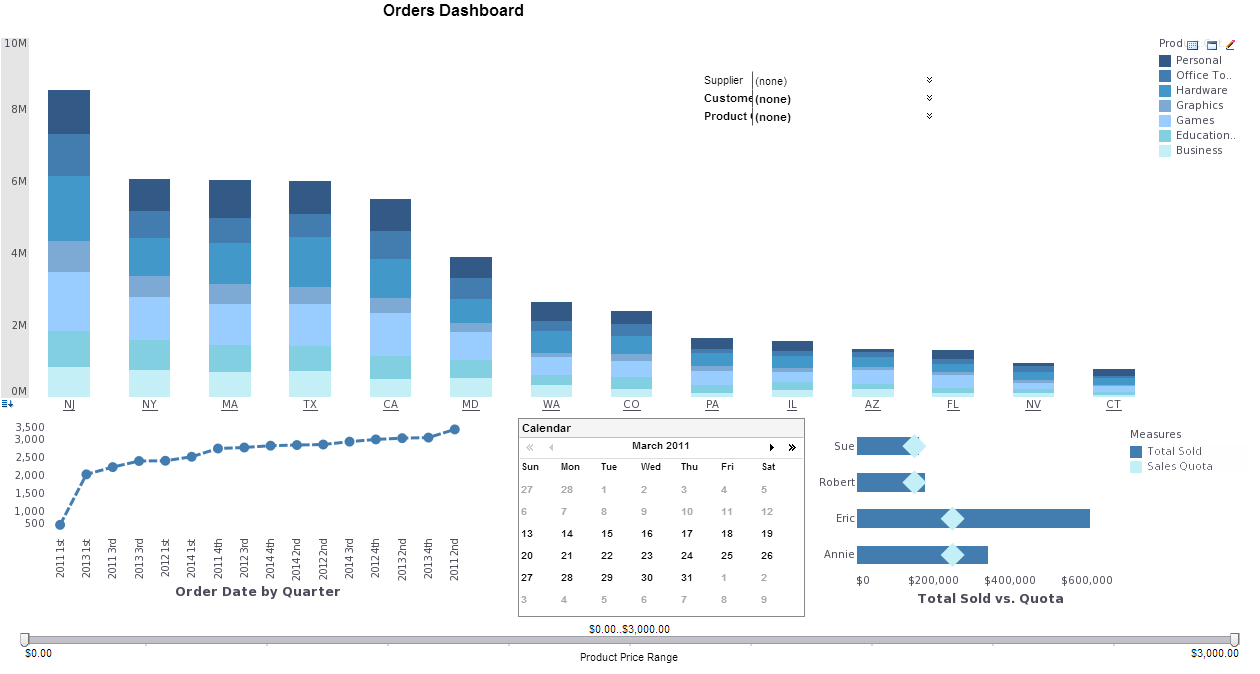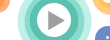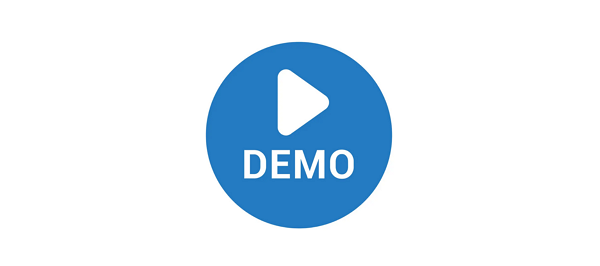# InetSoft Product Information: Top N Reports

After grouping we can further sort the table based on the section summarization. A Top N report will contain only the top 'n' sections (for example the top 3 results). This type of report is normally called a 'Top N' report. Top N table can only be generated on a table with grouping. Although it is possible to implement Top N filtering programmatically, the recommended way is to use the Report Designer GUI.

## Report Creation With Grouping/Summarization

We shall now create a report using the approach introduced in the Report Template-Based Programming chapter, in which grouping, summarization and Top N filtering are defined at the template level but raw data is retrieved and bound programmatically. We shall use the 'education.csv' file located in {InetSoftInstallation}/examples/docExamples/datasource/data to provide the raw data.

We shall start by creating the template in the Report Designer with File → New → Blank Tabular Report. Next, insert a table element with Insert → Basic Element → Table.

To add embedded data into a table, Right Click → Bind Data → Report → Embedded Data → Edit, set the number of rows to 2, the number of columns to 6 and the number of header rows to 1. Enter the column names in the following order (from left to right): State, School, Students, Type, Level, Province. Click 'OK' to continue.View a 2-minute demonstration of InetSoft's easy, agile, and robust BI software.

Click on the Grouping and Summary tab. Group by 'Type' and summarize by 'Students'. Select the grouping column 'Type' and add a Top N filter which will select the top 2 groups having the highest sum total of 'Students'.

Click the 'Finish' button and close the data binding window. Save the template as 'embeddedtable.srt'.

We shall now write a Java program which reads in the template as a ReportSheet object, loads the data file into a TableLens object, binds it to the table element and exports it as a PDF document.

```public boolean isPresenterOf(Class type) {
return Number.class.isAssignableFrom(type);
}```

Last, we define the paint() method for actually painting the bar. We can calculate the width of the bar using the value passed into paint() and the maximum value and presenter area width. Then we align the bar to the right of the area and paint.

```Builder bl = Builder.getBuilder(Builder.TEMPLATE,
new FileInputStream("embeddedtable.srt"));

TextTableLens ttl = new TextTableLens(
new FileInputStream("education.csv"),",");

rs.setElement("Table1", ttl);

Builder b = Builder.getBuilder(Builder.PDF,
new FileOutputStream("PDFOutput.pdf"));
b.write(rs);```View a 2-minute demonstration of InetSoft's easy, agile, and robust BI software.

## Formulas for Summarization

Formulas play a very important role in summarization processing. A formula decides how data is processed and summarized. InetSoft products include a number of built-in formulas that can be used directly to perform the common summarization functions, and Style Intelligence also provides an interface for adding user-defined formulas.

Table 7. Formulas in inetsoft.report.filter package

 Formula Description Sum Calculating the sum total of numbers. Average Calculating the average (mean) of numbers. Count Count the number of elements. Max Find the largest number. Min Find the smallest number. Distinct Count Calculate the number of distinct elements. Product Calculate the product (multiplication) of numbers. Standard Deviation Calculate the standard deviation of the number series. Variance Variance is a measure of dispersion. The mean of the square of the deviations is called the variance Population Variance This is the average squared distance between the mean and each item in the population. Population Standard Deviation Standard deviation is a measure of dispersion. It is the positive square root of the variance. See also variance. Correlation The correlation coefficient indicates the degree of linear relationship between two variables. The correlation coefficient always lies between -1 and +1. -1 indicates a perfect negative linear relationship between two variables, +1 indicates a perfect positive linear relationship and 0 indicates a lack of any linear relationship. Covariance The covariance between two random variables X and Y is the expected value of the product of the variables' deviations from their means. If there is a high probability that large values of X go with large values of Y and small values of X go with small values of Y, then the covariance between X and Y will be positive; if there is a high probability that small values of X go with large values of Y and large values of X go with small values of Y, then the covariance will be negative. Weighted Average A weighted average is a modified version of an arithmetic mean. An average of 5 and 7 is 12/2=6, but we can count 5 twice so that a weighted average is 17/3=5.67, etc. Median Calculates the value that is in the middle of a list. The median of a population is the point that divides the distribution of scores in half. pth Percentile The pth percentile of a data set is defined as that value where p percent of the data is below that value and (1-p) percent of the data is above that value. For example, the 50th percentile is the median. nth Largest Returns the first, second or nth largest number in a list. nth Smallest Returns the first, second or nth smallest number in a list. Mode Returns the value that occurs most frequently. nth Most Frequent Returns the first, second or nth most frequent value in a list. Concat Concatenates values into a comma-separated list.View live interactive examples in InetSoft's dashboard and visualization gallery.

### Summary of Top N Filtering

The most common data processing operation is the grouping and summarization of table rows. Grouping in Style Intelligence consists of two functions: grouping of rows based on one or more columns and summarization on the grouped sections. After grouping we can also provide top N filtering, which only displays a given number of values that are at the top or the bottom of the group. InetSoft products also include a number of built-in formulas that can be used directly to perform the common summarization functions. We also discussed in detail the recommended report creation technique using an approach in which grouping, summarization and Top N filtering are defined at the template level but raw data is retrieved and bound programmatically.

 Previous: Grouping and Summarization of Tabular Data